oscilloscope, you would see continuous square or rectangular waveforms. The astable multivibrator has two outputs, but NO inputs. ">Custom SearchAstable Multivibrator An astable multivibrator is also known as a FREE-RUNNING MULTIVIBRATOR. It is called free-running because it alternates between two different output voltage levels during the time it is on. The output remains at each voltage level for a definite period of time. If you looked at this output on an oscilloscope, you would see continuous square or rectangular waveforms. The astable multivibrator has two outputs, but NO inputs. Let's look at the multivibrator in figure 3-3 again. This is an astable multivibrator. The astable multivibrator is said to oscillate. To understand why the astable multivibrator oscillates, assume that transistor Q1 saturates and transistor Q2 cuts off when the circuit is energized. This situation is shown in figure 3-4. We assume Q1 saturates and Q2 is in cutoff because the circuit is symmetrical; that is, R1 = R4, R2 = R3, C1 = C2, and Q1 = Q2. It is impossible to tell which transistor will actually conduct when the circuit is energized. For this reason, either of the transistors may be assumed to conduct for circuit analysis purposes. Figure 3-4. - Astable multivibrator (Q1 saturated).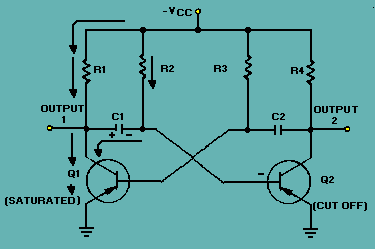Essentially, all the current in the circuit flows through Q1; Q1 offers almost no resistance to current flow. Notice that capacitor C1 is charging. Since Q1 offers almost no resistance in its saturated state, the rate of charge of C1 depends only on the time constant of R2 and C1 (recall that TC = RC). Notice that the right-hand side of capacitor C1 is connected to the base of transistor Q2, which is now at cutoff. Let's analyze what is happening. The right-hand side of capacitor C1 is becoming increasingly negative. If the base of Q2 becomes sufficiently negative, Q2 will conduct. After a certain period of time, the base of Q2 will become sufficiently negative to cause Q2 to change states from cutoff to conduction. The time necessary for Q2 to become saturated is determined by the time constant R2C1. The next state is shown in figure 3-5. The negative voltage accumulated on the right side on capacitor C1 has caused Q2 to conduct. Now the following sequence of events takes place almost instantaneously. Q2 starts conducting and quickly saturates, and the voltage at output 2 changes from approximately -VCC to approximately 0 volts. This change in voltage is coupled through C2 to the base of Q1, forcing Q1 to cutoff. Now Q1 is in cutoff and Q2 is in saturation. This is the circuit situation shown in figure 3-6. Figure 3-5. - Astable multivibrator.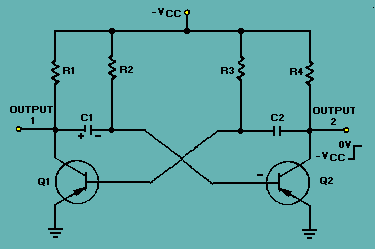Figure 3-6. - Astable multivibrator. (Q2 saturated).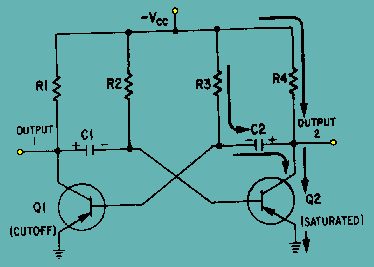Notice that figure 3-6 is the mirror image of figure 3-4. In figure 3-6 the left side of capacitor C2 becomes more negative at a rate determined by the time constant R3C2. As the left side of C2 becomes more negative, the base of Q1 also becomes more negative. When the base of Q1 becomes negative enough to allow Q1 to conduct, Q1 will again go into saturation. The resulting change in voltage at output 1 will cause Q2 to return to the cutoff state. Look at the output waveform from transistor Q2, as shown in figure 3-7. The output voltage (from either output of the multivibrator) alternates from approximately 0 volts to approximately -VCC, remaining in each state for a definite period of time. The time may range from a microsecond to as much as a second or two. In some applications, the time period of higher voltage (-VCC) and the time period of lower voltage (0 volts) will be equal. Other applications require differing higher- and lower-voltage times. For example, timing and gating circuits often have different pulse widths as shown in figure 3-8. Figure 3-7. - Square wave output from Q2.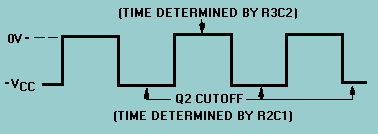Figure 3-8. - Rectangular waves.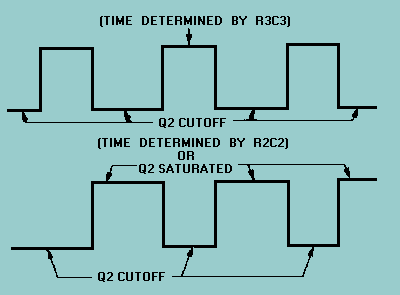FREQUENCY STABILITY. - Some astable multivibrators must have a high degree of frequency stability. One way to obtain a high degree of frequency stability is to apply triggers. Figure 3-9, view (A), shows the diagram of a triggered, astable multivibrator. At time T0, a negative input trigger to the base of Q1 (through C1) causes Q1 to go into saturation, which drives Q2 to cutoff. The circuit will remain in this condition as long as the base voltage of Q2 is positive. The length of time the base of Q2 will remain positive is determined by C3, R3, and R6. Observe the parallel paths for C3 to discharge. Figure 3-9A. - Triggered astable multivibrator and output.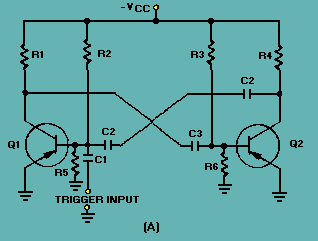View (B) of figure 3-9 shows the waveforms associated with the circuit. At time T1, Q2 comes out of cutoff and goes into saturation. Also, Q1 is caused to come out of saturation and is cut off. The base voltage waveform of Q1 shows a positive potential that is holding Q1 at cutoff. This voltage would normally hold Q1 at cutoff until a point between T2 and T3. However, at time T2 another trigger is applied to the base of Q1, causing it to begin conducting. Q1 goes into saturation and Q2 is caused to cut off. This action repeats each time a trigger (T2, T4, T6) is applied. Figure 3-9B. - Triggered astable multivibrator and output.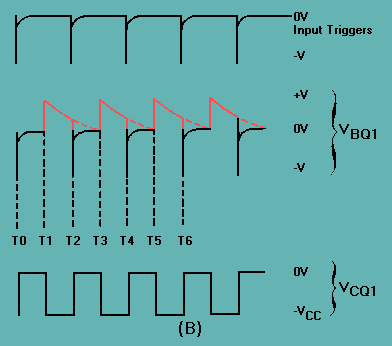The prt of the input triggers must be shorter than the natural free-running prt of the astable multivibrator, or the trigger prf must be slightly higher than the free-running prf of the circuit. This is to make certain the triggers control the prt of the output. Monostable Multivibrator The monostable multivibrator (sometimes called a ONE-SHOT MULTIVIBRATOR) is a square- or rectangular-wave generator with just one stable condition. With no input signal (quiescent condition) one amplifier conducts and the other is in cutoff. The monostable multivibrator is basically used for pulse stretching. It is used in computer logic systems and communication navigation equipment. The operation of the monostable multivibrator is relatively simple. The input is triggered with a pulse of voltage. The output changes from one voltage level to a different voltage level. The output remains at this new voltage level for a definite period of time. Then the circuit automatically reverts to its original condition and remains that way until another trigger pulse is applied to the input. The monostable multivibrator actually takes this series of input triggers and converts them to uniform square pulses, as shown in figure 3-10. All of the square output pulses are of the same amplitude and time duration.Integrated Publishing, Inc. - A (SDVOSB) Service Disabled Veteran Owned Small Business